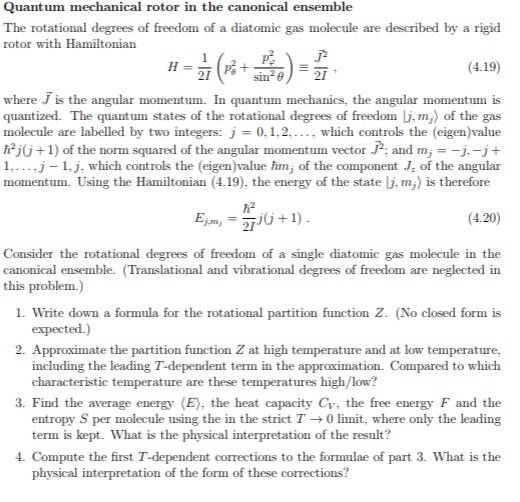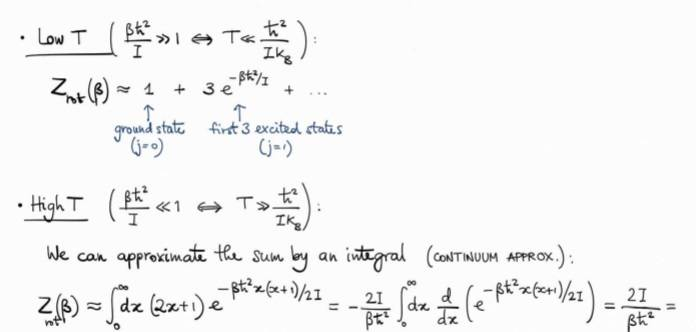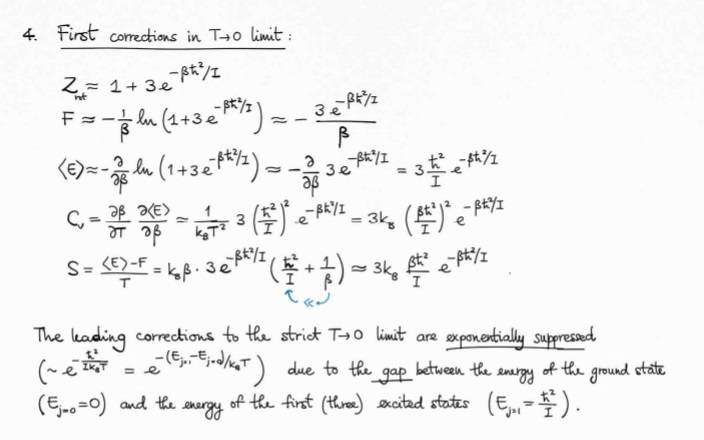# Statistical Mechanics-Limit in canonical ensemble

binbagsss

## Homework Statement

question attached.My question is just about the size of the limit, how do you know whether to expand out the exponential or not (parts 2) and 4))

## Homework Equations

for small ##x## we can expand out ##e^{x} ## via taylor series.

## The Attempt at a Solution

Solutions given here for parts 2) and 4) respectively. (not full sol but only as relevant as needed for the question):For parts 2) and 4) below I would have used the same approximation ##1+3e^{x}##, but would have expanded out the exponential as ##1+x##.

I'm just wondering how you know whether or not to expand this out or not, going by 'low ##T## many thanks.

#### Attachments

Homework Helper
Gold Member
For parts 2) and 4) below I would have used the same approximation ##1+3e^{x}##, but would have expanded out the exponential as ##1+x##.

I'm just wondering how you know whether or not to expand this out or not, going by 'low ##T## many thanks.
The approximation ##e^x \approx 1 + x## is only valid if ##x## is "small" (i.e., ##|x| \ll 1##).

For low ##T##, is the argument of the exponential small in any of the terms of ##Z## (other than the ##j = 0## term)?

binbagsss
The approximation ##e^x \approx 1 + x## is only valid if ##x## is "small" (i.e., ##|x| \ll 1##).

For low ##T##, is the argument of the exponential small in any of the terms of ##Z## (other than the ##j = 0## term)?

would you not have to know an approx magnitude of the moment of inertia to know this? or should we be looking at planks constant, and Boltzmann constant, and physically feasible magnitudes of the moment of inertia, i hae no idea what these would be , and know they arent comparable so x is not small?

Homework Helper
Gold Member
The exponential factors in ##Z## have the form ##e^{-\beta E_j}##, where ##\beta E_j = \frac{\hbar^2 j(j+1)}{2 I k T}##.
As ##T## →0, ##\beta E_j## gets large (for any ##j > 0##). So, ##e^{-\beta E_j}## → 0 for any for any ##j > 0##. The approximation ##e^x \approx 1+x## doesn't apply here. The larger the value of ##j##, the faster ##e^{-\beta E_j}## → 0. So, as an approximation, you can keep just the ##j = 0## and ##j = 1## terms in ##Z##.